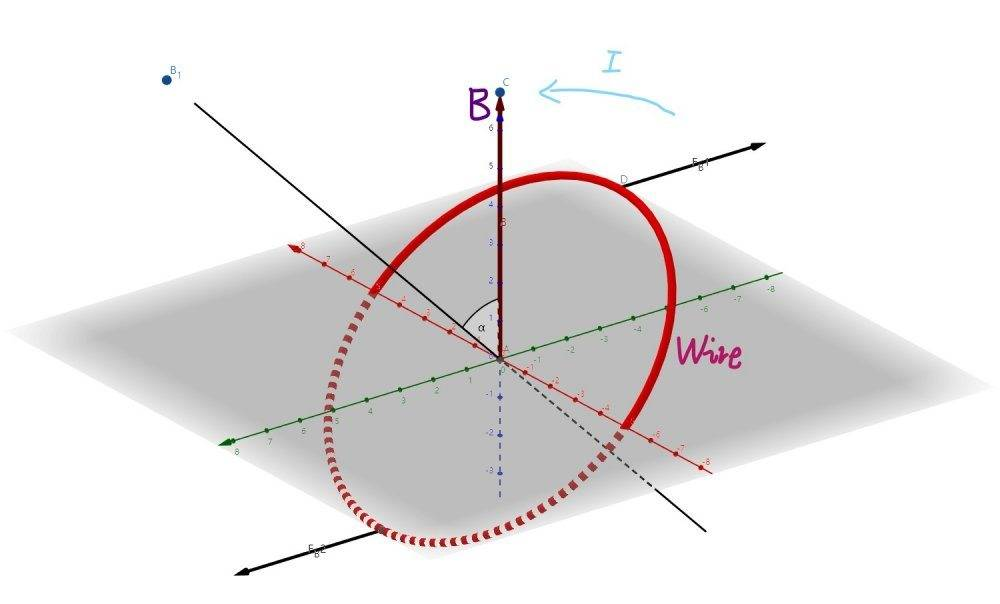# Torque on a circular current loop

Homework Statement:
This is a conceptual question.
Relevant Equations:
$$\vec{\tau}=I \vec{A} \times \vec{B}$$
Can anyone please tell me why the torque on a circular current loop can be calculated by the equation below? In other words, what is the intuition for the formula? Thank you. (I modified my question to a particular case)
$$\vec{\tau} = I \vec{A} \times \vec{B}$$

Last edited:

BvU
Homework Helper
Try to figure it out starting from the Lorentz force
Hi. I have updated my question. I have not learned vector calculus since I am in high school and this is how far I can go.

$$d\vec{F} = I d\vec{L} \times \vec{B}$$
$$\vec{r} \times d\vec{F} = \vec{r} \times (I d\vec{L} \times \vec{B})$$
$$d\tau = \vec{r} \times (I d\vec{L} \times \vec{B})$$
$$\int d\tau = \int ((\vec{r} \times I d\vec{L}) \times \vec{B})$$
$$\int d\tau = \int rI d(L\hat{n} \times \vec{B})$$

haruspex
Homework Helper
Gold Member
2020 Award
Thanks, but I do understand the case in which the wire is rectangular. I would like to know why the formula is still valid when the loop is circular.

BvU
Homework Helper
Go back to the Lorentz force and do the calculation.

Hey, didn't I reply something similar earlier on ?

kuruman
Homework Helper
Gold Member
Actually the torque is ##\vec r \times \vec F~\rightarrow~\vec \tau = \int \vec r \times I (d\vec L \times \vec B)=I\int \vec r \times (d\vec L \times \vec B)##. The question of an arbitrary shaped current loop is normally treated in an intermediate-level EM textbok. I will not reproduce the solution here in favor of a hand-waving argument that might satisfy you because it is a description of the mathematical argument that adds and subtracts a whole bunch of zeroes.

Suppose you subdivide an arbitrary shaped loop carrying current ##I## into many infinitesimally small rectangular loops that fill the area of the original loop. Each of these loops carries current ##I## determined by the a right hand rule consistent with the normal ##\hat n## to the loop. The ##i##th loop contributes torque ##d\vec \tau_i=d\vec m_i \times \vec B=I dA_i~\hat n \times \vec B##. Adding all such contributions gives the total torque ##\vec \tau=I A~\hat n \times \vec B##.

Note that any rectangular loop that has a common boundary with another loop can be combined into a larger loop because current in the common boundary runs in opposite directions and therefore has no contribution to the net force or the net torque. The contribution to the torque of both loops together would be ##d\vec \tau_{i,i+1}=d\vec m_{i,i+1} \times \vec B=I (dA_i+dA_{i+1})~\hat n \times \vec B## and so on until one runs out of neighboring loops having reached the boundary.

Thus the internal common boundaries can be ignored and what's left is the outside boundary of the arbitrary loop that contributes to the total torque and that has already been found.

••Leo Liu and etotheipi
etotheipi
Note that any rectangular loop that has a common boundary with another loop can be combined into a larger loop because current in the common boundary runs in opposite directions and therefore has no contribution to the net force or the net torque. The contribution to the torque of both loops together would be ##d\vec \tau_{i,i+1}=d\vec m_{i,i+1} \times \vec B=I (dA_i+dA_{i+1})~\hat n \times \vec B## and so on until one runs out of neighboring loops having reached the boundary.

Thus the internal common boundaries can be ignored and what's left is the outside boundary of the arbitrary loop that contributes to the total torque and that has already been found.

Wow, that's such a cool approach! I've been messing around with horrible integrals and this is much nicer!

Suppose you subdivide an arbitrary shaped loop carrying current ##I## into many infinitesimally small rectangular loops that fill the area of the original loop. Each of these loops carries current ##I## determined by the a right hand rule consistent with the normal ##\hat n## to the loop. The ##i##th loop contributes torque ##d\vec \tau_i=d\vec m_i \times \vec B=I dA_i~\hat n \times \vec B##. Adding all such contributions gives the total torque ##\vec \tau=I A~\hat n \times \vec B##.